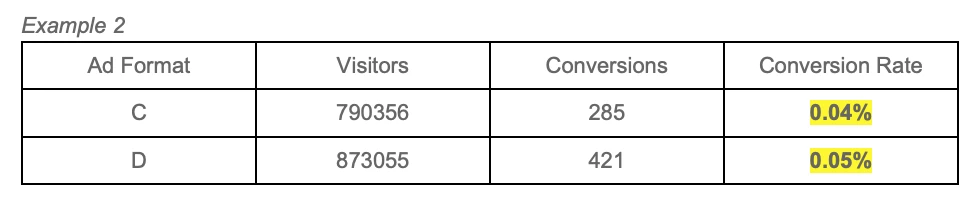Search

# The significance of being statistically significant in campaign optimization

You may think your ad is doing well, but there’s a catch...As marketers, we often need to evaluate ad performance across different ads through A/B testing. However, by simply comparing the result may not be accurate in finding out which ads work well. For example, in the table below:Ad A has conversion rate of 1% while Ad B has conversion rate of 0.8%. By instinct, we would think that Ad A is doing better than B (1% > 0.8%), and proceed to use Ad A for rest of the campaign. Is this true? By applying statistical significance testing, it turns out that it’s not conclusive to determine that Ad A has better performance than Ad B. So, how can we make sure we are making the right decision on which ad to pause? First, let’s find out what statistical significance is.

What it means to be statistically significance

Statistical significance is the likelihood that the difference in conversion rates between a given variation and the baseline is not due to random chance (Optimizely, 2021). So how do we calculate and compare which ad is better? There are different levels of statistical significance using confidence levels: 90%, 95%, and 99%. For example, using 95% confidence level reflects that we are 95% confident that the result is correct without random error. On the flip side, this can also mean that there is a 5% chance that the result could be wrong.

In statistical significance, we also use p-value. The p-value, also known as probability value, is a number describing how likely it is that the data would have occurred by random chance (Saul, 2019). P-value number is typically 0.1, 0.05, and 0.01. In this study, our benchmark will be 0.05. Thus, if the p-value is less than 0.05, the result is significant. To make sure that the ad does have a better performance, we have to use statistical significance to prove that our hypothesis is right.

How to compare data?

Let’s look at the first example.There is an easy way to get the result for statistical significance using an A/B testing calculator. Here is an example of the calculator that we will be using for this exercise. It is simple to use as all you have to do is key in the information and the calculator will generate the result for conversion rate and the significance of the different confidence level with explanation.Here, p-value is 0.8995. To determine a result is significant, p-value < 0.05. Thus, the result concluded that with 90%,95% and 99% confidence, the result is not significant because there is not enough evidence that the difference in groups is not due to chance.

Therefore, we cannot say that A is performing better than B, nor can we say that B will perform worse than A.

It is best to have both ad formats run for another week and observe if there will be a change.

Let's look at another example:Based on the conversion rate, D is performing better. Let’s check for the significance.At all the confidence levels, the result is significant that ad D has better performance with 0.05% conversion rate than ad C with 0.04% conversion rate. Furthermore, the p-value is less than 0.05. This means that we are very much confident that D will perform better than C.

This way, we can say that ad D is more effective in driving conversion and that we can recommend stopping ad C.

Which Confidence Levels should we use?

The higher the confidence level, the more accurate the result will be, however, there is usually a trade-off between accuracy, and the number of sample sizes require to get to statistical significance. In the research world, the most commonly used confidence level is 95% (Source: Evolytics), though our recommendation is that in the interest of time (Testing period), a confidence level of 90% will suffice.

Conclusion

To determine which ad will perform better, we cannot simply “look” and use our gut feelings. By using statistical significance, we can determine more accurately which ad performs better.

Thus, based on the examples we went through, statistical significance matters more than the duration of the campaign. However, you do need to run the campaign long enough to get enough evidence. So, if it seems like the result is not significant, you want to run the test for a few more weeks to generate enough sample size before concluding. Conversely for ads that are performing well in the beginning, if you conclude too fast, it may appear to have a significant difference at first, but this difference may actually decrease over time.

We help advertisers go beyond A/B testing and optimize your ads in real-time using machine learning algorithms. Our proprietary optimization engine takes away the guesswork, analyzes each impression and selects the best ads to be served. We will share more about this in an upcoming blog post.